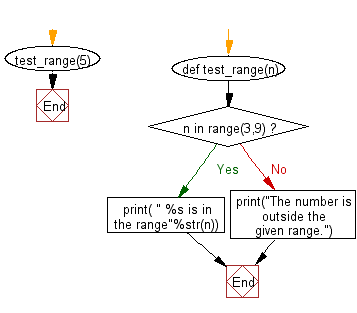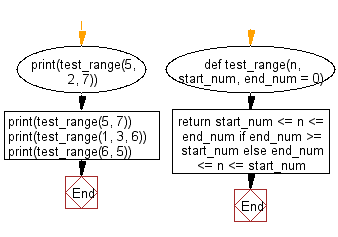﻿ Python Exercise: Check whether a number falls in a given range - w3resource# Python Exercise: Check whether a number falls in a given range

## Python Functions: Exercise-6 with Solution

Write a Python function to check whether a number falls in a given range.

Sample Solution-1:

Python Code:

``````def test_range(n):
if n in range(3,9):
print( " %s is in the range"%str(n))
else :
print("The number is outside the given range.")
test_range(5)
```
```

Sample Output:

``` 5 is in the range
```

Pictorial presentation:Flowchart:## Visualize Python code execution:

The following tool visualize what the computer is doing step-by-step as it executes the said program:

Sample Solution-2:

• Use arithmetic comparison to check if the given number is in the specified range.
• If the second parameter, end, is not specified, the range is considered to be from 0 to start.

Python Code:

``````def test_range(n, start_num, end_num = 0):
return start_num <= n <= end_num if end_num >= start_num else end_num <= n <= start_num
print(test_range(5, 2, 7))
print(test_range(5, 7))
print(test_range(1, 3, 6))
print(test_range(6, 5))
```
```

Sample Output:

```True
True
False
False
```

Flowchart:## Visualize Python code execution:

The following tool visualize what the computer is doing step-by-step as it executes the said program:

Python Code Editor:

Have another way to solve this solution? Contribute your code (and comments) through Disqus.

What is the difficulty level of this exercise?

Test your Programming skills with w3resource's quiz.

﻿

## Python: Tips of the Day

Decapitalizes the first letter of a string:

Example:

```def tips_decapitalize(s, upper_rest=False):
return s[:1].lower() + (s[1:].upper() if upper_rest else s[1:])
print(tips_decapitalize('PythonTips'))
print(tips_decapitalize('PythonTips', True))
```

Output:

```pythonTips
pYTHONTIPS
```